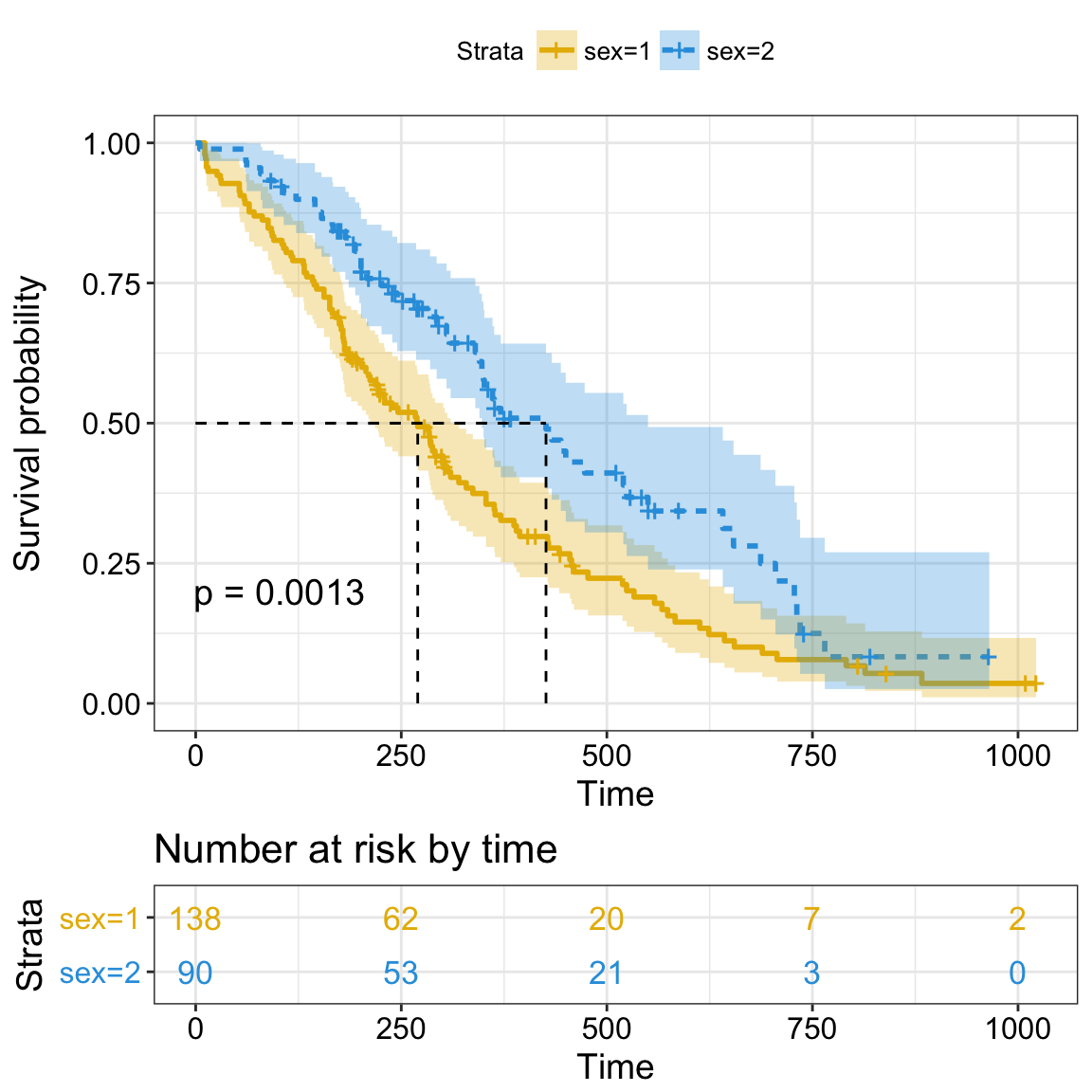# Survival Analysis

Survival analysis corresponds to a set of statistical methods for investigating the time it takes for an event of interest to occur.

In this chapter, we start by describing how to fit survival curves and how to perform logrank tests comparing the survival time of two or more groups of individuals. We continue by demonstrating how to assess simultaneously the impact of multiple risk factors on the survival time using the Cox regression model. Finally, we describe how to check the validy Cox model assumptions.

## Survival analysis toolkits in R

We’ll use two R packages for survival data analysis and visualization :

1. the survival package for survival analyses,
2. and the survminer package for ggplot2-based elegant visualization of survival analysis results

For survival analyses, the following function [in survival package] will be used:

• Surv() to create a survival object
• survfit() to fit survival curves (Kaplan-Meier estimates)
• survdiff() to perform log-rank test comparing survival curves
• coxph() to compute the Cox proportional hazards model

For the visualization, we’ll use the following function available in the survminer package:

• ggsurvplot() for visualizing survival curves
• ggcoxzph(), ggcoxdiagnostics() and ggcoxfunctional() for checking the Cox model assumptions.

These two packages can be installed as follow:

``````install.packages("survival")
install.packages("survminer")``````

## Survival analysis basics: curves and logrank testsSurvival analysis

• Objectives
• Basic concepts
• Survival time and type of events in cancer studies
• Censoring
• Survival and hazard functions
• Kaplan-Meier survival estimate
• Survival analysis in R
• Install and load required R package
• Example data sets
• Compute survival curves: survfit()
• Visualize survival curves
• Kaplan-Meier life table: summary of survival curves
• Log-Rank test comparing survival curves: survdiff()
• Fit complex survival curves

## Cox proportional hazards model

• The need for multivariate statistical modeling
• Basics of the Cox proportional hazards model
• Compute the Cox model in R
• Install and load required R package
• R function to compute the Cox model: coxph()
• Example data sets
• Compute the Cox model
• Visualizing the estimated distribution of survival times

Read more –> Cox Proportional Hazards Model.

## Cox model assumptions

• Diagnostics for the Cox model
• Assessing the validy of a Cox model in R
• Computing a Cox model
• Testing proportional Hazards assumption
• Testing influential observations
• Testing non linearity

Read more –> Cox Model Assumptions.

## Infos

This analysis has been performed using R software (ver. 3.3.2).